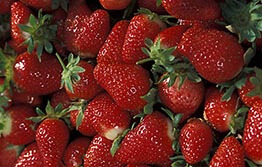```int(43)
bool(false)
int(0)
```
```int(1306)
bool(false)
int(43)
```
```int(2309)
bool(false)
int(43)
```
```int(55)
bool(false)
int(43)
```
```int(1307)
bool(false)
int(43)
```
```int(41)
bool(false)
int(0)
```
```int(60)
bool(false)
int(41)
```
```int(1311)
bool(false)
int(41)
```
```int(1371)
bool(false)
int(41)
```
```int(2576)
bool(false)
int(41)
```
```int(406)
bool(false)
int(41)
```
```int(42)
bool(false)
int(0)
```
```int(1304)
bool(false)
int(42)
```
```int(1303)
bool(false)
int(42)
```
```int(407)
bool(false)
int(42)
```
```int(62)
bool(false)
int(42)
```
```int(51)
bool(false)
int(42)
```
```int(2587)
bool(false)
int(42)
```
```int(3161)
bool(false)
int(42)
```
```int(3163)
bool(false)
int(42)
```
```int(3164)
bool(false)
int(42)
```
```int(3165)
bool(false)
int(42)
```
```int(3166)
bool(false)
int(42)
```
```int(3167)
bool(false)
int(42)
```
```int(3168)
bool(false)
int(42)
```
```int(3169)
bool(false)
int(42)
```
```int(3170)
bool(false)
int(42)
```
```int(3171)
bool(false)
int(42)
```
```int(3172)
bool(false)
int(42)
```
```int(3173)
bool(false)
int(42)
```
```int(3174)
bool(false)
int(42)
```
```int(2951)
bool(false)
int(0)
```
```int(2952)
bool(false)
int(2951)
```
```int(2953)
bool(false)
int(2951)
```
```int(2954)
bool(false)
int(2951)
```
```int(2955)
bool(false)
int(2951)
```

## Strawberry trade from WA okay again

Biosecurity Tasmania advises that new, temporary import requirements have been imposed on the trade of Western Australian strawberry fruit as a vector of green snail Cornu apertus (Born) (syn. Cantareus apertus (Born), Helix aperta (Born).

As a result, strawberries from properties more than two kilometres from a green snail infestation in Western Australia can once again be imported into Tasmania in accordance with a series of import conditions, including that properties must be inspected free of the pest by an approved person on a monthly basis.Strawberry fruit from Western Australian properties within two kilometres of a green snail infestation will still not be accepted for the time being.

Biosecurity Tasmania continues to work with our Western Australian counterparts on this matter.